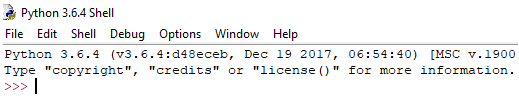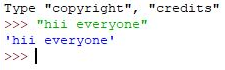Input and Output

# Input and Output Notes - Class 9

## Document Description: Input and Output for Class 9 2022 is part of Input/Output for preparation. The notes and questions for Input and Output have been prepared according to the Class 9 exam syllabus. Information about Input and Output covers topics like and Input and Output Example, for Class 9 2022 Exam. Find important definitions, questions, notes, meanings, examples, exercises and tests below for Input and Output.

Introduction of Input and Output in English is available as part of our for Class 9 & Input and Output in Hindi for course. Download more important topics related with Input/Output, notes, lectures and mock test series for Class 9 Exam by signing up for free. Class 9: Input and Output Notes - Class 9
 1 Crore+ students have signed up on EduRev. Have you?

What is Console in Python?
Console (also called Shell) is basically a command line interpreter that takes input from the user i.e one command at a time and interprets it. If it is error free then it runs the command and gives required output otherwise shows the error message. A Python Console looks like this.Here we write command and to execute the command just press enter key and your command will be interpreted.
For coding in Python you must know the basics of the console used in Python.
The primary prompt of the python console is the three greater than symbols

>>>

You are free to write the next command on the shell only when after executing the first command these prompts have appeared. The Python Console accepts command in Python which you write after the prompt.Accepting Input from Console
User enters the values in the Console and that value is then used in the program as it was required.
To take input from the user we make use of a built-in function input().

# input

input1 = input()

# output

print(input1)

We can also type cast this input to integer, float or string by specifying the input() function inside the type.

• Typecasting the input to Integer: There might be conditions when you might require integer input from user/Console, the following code takes two input(integer/float) from console and typecasts them to integer then prints the sum.

`# input`
`num1 ``=` `int``(``input``())`
`num2 ``=` `int``(``input``())`
` `
`# printing the sum in integer`
`print``(num1 ``+` `num2)`

• Typecasting the input to Float: To convert the input to float the following code will work out.

# input

num1 = float(input())

num2 = float(input())

# printing the sum in float

print(num1 + num2)

• Typecasting the input to String: All kind of input can be converted to string type whether they are float or integer. We make use of keyword str for typecasting.

# input

string = str(input())

# output

print(string)

How to Input Multiple Values From User In One Line
For instance, in C we can do something like this:

// Reads two values in one line

scanf("%d %d", &x, &y)

One solution is to use raw_input() two times.

x, y = input(),  input()

Another solution is to use split()

x, y = input().split()

Note that we don’t have to explicitly specify split(‘ ‘) because split() uses any whitespace characters as a delimiter as default.
One thing to note in the above Python code is, both x and y would be of string. We can convert them to int using another line.

x, y = [int(x), int(y)]

# We can also use  list comprehension

x, y = [int(x) for x in [x, y]]

Below is complete one line code to read two integer variables from standard input using split and list comprehension

# Reads two numbers from input and typecasts them to int using

# list comprehension

x, y = [int(x) for x in input().split()]

# Reads two numbers from input and typecasts them to int using

# map function

x, y = map(int, input().split())

END Parameter
By default python’s print() function ends with a newline. A programmer with C/C++ background may wonder how to print without newline.
Python’s print() function comes with a parameter called ‘end’. By default, the value of this parameter is ‘\n’, i.e. the new line character. You can end a print statement with any character/string using this parameter.

# This Python program must be run with

# Python 3 as it won't work with 2.7.

# ends the output with a <space>

print("Welcome to" , end = ' ')

print("GeeksforGeeks", end = ' ')

Output :

Welcome to GeeksforGeeks

One more program to demonstrate working of end parameter.

# This Python program must be run with

# Python 3 as it won't work with 2.7.

# ends the output with '@'

print("Python" , end = '@')

print("GeeksforGeeks")

Output :

Python@GeeksforGeeks

The document Input and Output Notes - Class 9 is a part of Class 9 category.
All you need of Class 9 at this link: Class 9
 Use Code STAYHOME200 and get INR 200 additional OFF

Track your progress, build streaks, highlight & save important lessons and more!

,

,

,

,

,

,

,

,

,

,

,

,

,

,

,

,

,

,

,

,

,

;